• 我们都知道计算机是以二进制的方式来处理各项运算的，有时候还会用到八进制，十六进制数，而我们人类则是以十进制的方式来处理数据和信息的...十进制数字转换成二进制十进制数字转换成八进制： 十进制数字转换成十
我们都知道计算机是以二进制的方式来处理各项运算的，有时候还会用到八进制，十六进制数，而我们人类则是以十进制的方式来处理数据和信息的（当然，有没有大神以其他的进制来处理我们就不知道了。）

二进制数字：0，1

八进制数字：0，1，2，3，4，5，6，7

十进制数字：0，1，2，3，4，5，6，7，8，9

十六进制数字：0，1，2，3，4，5，6，7，8，9，A（10），B（11），C（12），D（13），E（14），F（15）

十进制数字转换成二进制：

例如：（65.325）10 = （1000001.011）2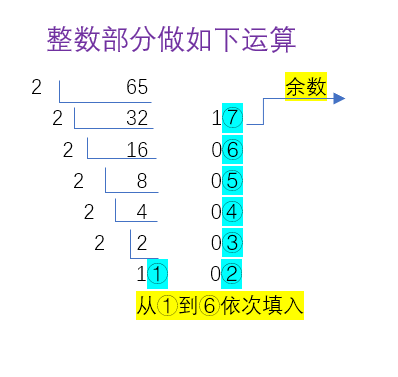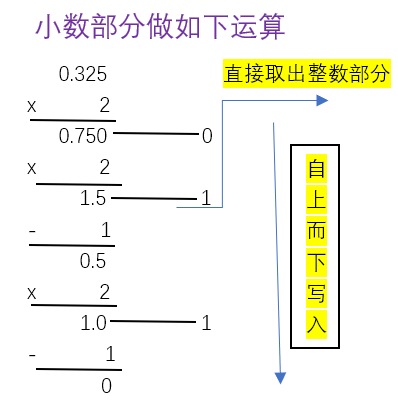十进制数字转换成八进制：

例如：（86.35）10 = （126.263）8  注： 一般小数部分取前三位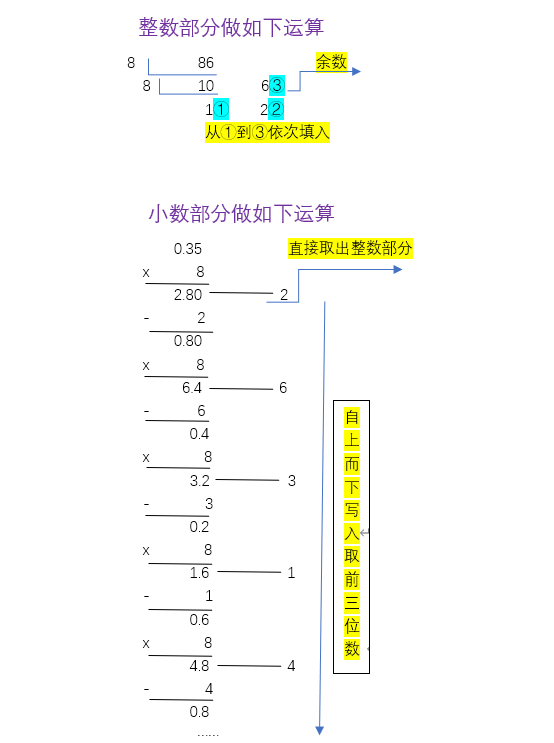十进制数字转换成十六进制：

例如：（140.95）10 = （8C.F33)16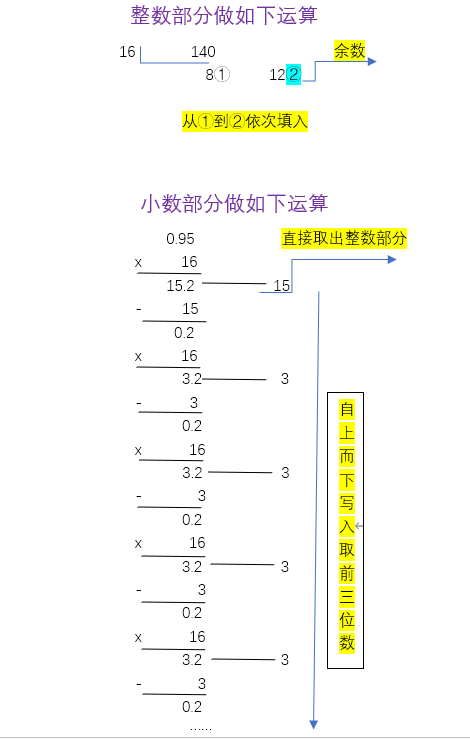规律：十进制转换成相应进制的数字，整数部分除以对应进制取余，在除以进制在取余，依次计算下去，然后按照从下到上而写；小数部分则是乘以对应进制取整数，然后依次再乘以进制数，再取整数，有的可能无限位数，则取前三位，由上到下！

二进制准换成十进制：

例如：（111000.011）2 = （56.375）10

对应位数的数字乘以相应的2的对应次幂，个位数起始为2的0次幂，整数从右往左算起，小数部分为2的负次幂乘以对应的分位数（十分位为2^-1)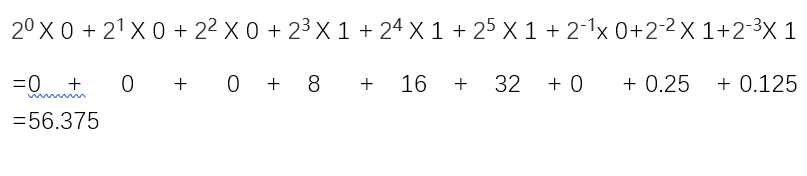二进制转换成八进制：

例如：（111000.011）2 = （70.3）8

111  = 2^2 X 1 + 2^1 X 1 + 2^0 X 1 = 7

000 = 2^2 X 0 + 2^1 X 0 + 2^0 X 0 = 0

011 = 2^2 X 0 + 2^1 X 1 + 2^0 X 1  = 3

学名叫做三合一法，就是从小数点开始，左面每三个为一组，不足三位用零补齐，小数点右面也是每三个一组，不足用零补齐。三个为一组用2的对应次幂乘以对应的数，从右往左依次是2^0，2^1，2^2 小数部分也是从右往左算。

八进制转换成二进制：

逆运算就可以了，每一位数字拆开写，然后按照4（2^2) 2（2^1） 1（2^0) 的位权进行相加 ，哪个符合哪位就是1 不符合的数字就是0

比如上面的例子：（70.3）8  = （111000.011）2

7 =  4 + 2 + 1 = （111）2

0 =  0 + 0 + 0 =  （000）2

3 = 0  + 2 + 1 = （011）2

二进制转换成十六进制：

四合一法，和转换八进制类似，只不过是四个为一组，不足用零补齐，高位是2^3，低位是2^0,小数部分也是如此

例如：（111000.011）2 = （38.6）16

0011（不足用0补齐，熟练了就不用了，自己心中有数） = 2^3 X0 + 2^2 X 0 +2^1 X 1 + 2^0 X 1 = 3

1100                                                                                  =2^3 X1 + 2^2 X 0 +2^1 X 0 + 2^0 X 0  = 8

0110（小数部分建议补齐，有时候容易出错）                 =2^3 X0 + 2^2 X 1 +2^1 X 1 + 2^0 X 0  = 6

十六进制转换成二进制：

也是逆运算，每个数字拆成四位二进制数，位权从高到低依次为8 4 2 1  符合的为1  不符合的为 0

比如上面的例子：（38.6）16  = （111000.011）2  对应的零可以省略

3 = 0 + 0 + 2 + 1 = （0011）2

8 = 8 + 0 + 0 + 0 =  （1000）2

6 = 0 + 4 + 2 + 0 =  （0110）2

八进制转成十进制：

和二进制转十进制的方法类似，因为是八进制，所以对应的位权就是8的相应次幂，算法就是对应位数的数字乘以对应的位权。

例如：（94.25）8 = （76.328125）10

9 x 8^1 + 4 x 8^0  +  2 x 8^-1  + 5 x 8^-2  = 72 + 4 + 0.25 + 0.078125 = 76.328125

十六进制转换成十进制：

和其他进制转成十进制类似，只不过位权是16的相应次幂，算法也是对应位数的数字乘以对应的位权

例如：（FA.BC）16 =  （250.734375）10

15 x 16^1 + 10 x  16^0  + 11 x 16^-1  + 12 x 16^-2  =  240 + 10 + 0.6875 + 0.046875 = 250.734375

八进制转换成十六进制 和 十六进制转换成八进制 均以二进制为中介或者也可以十进制为中介

展开全文算法
• 1、定义 16进制，英文名简写为hex，是一种逢十六进一的进位制，会...十进制转十六进制：除以16，余数为最低一位，商再除以16得余数为倒数第位，直到商为0. 举例：将27804转换十六进制 27804/16=1737…12(C ) 17
1、定义

16进制，英文名简写为hex，是一种逢十六进一的进位制，会用到数字0~9和字母A至F（或a至f）表示。与十进制对应的结果如下：

十六进制
0
1
2
3
4
5
6
7
8
9
A
B
C
D
E
F

十进制
0
1
2
3
4
5
6
7
8
9
10
11
12
13
14
15

2、具体换算（人工）
十进制转十六进制：除以16，余数为最低一位，商再除以16得余数为倒数第二位，直到商为0.
举例：将27804转换成十六进制
27804/16=1737…12(C )
1727/16=108…9(9)
108/16=6…12(C )
6/16=0…6(6)
所以27804转换成16进制最后的结果是  6C9C
3、用代码实现
>>>hex(27804)
'0x6c9c'


展开全文• 比如二进制1101，知换算成十进制就是：1*2（1-1）+0*2（2-1）+1*2（3-1）+1*2（4-1）=1+0+4+8=13。 扩展资道料： 1、二进制转换为八进制： 把二进制从右往左，三位一组，不够补0 列：111=4+2+1=7 11001拆
从最低位（最右）算起，位上的数字乘以本位的权重，权重就百是2的第几位的位数减一次方。

比如第2位就是2的（2-1次）方，就是2；第8位就是2的（8-1）次方是128。把所有度的值加起来。

2（1-1）代表2的0次方，就是1；其他类推

比如二进制1101，知换算成十进制就是：1*2（1-1）+0*2（2-1）+1*2（3-1）+1*2（4-1）=1+0+4+8=13。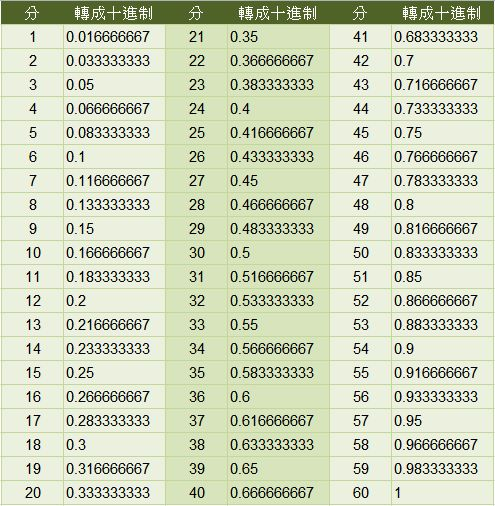扩展资道料：

1、二进制转换为八进制：

把二进制的数从右往左，三位一组，不够补0

列：111=4+2+1=7

11001拆分为 001和011，001=1，011=2+1=3。

那么11001转换为八进制就是31。

2、二进制转换为十六进制：

参照二进制转八内进制，但是它是从右往左，四位一组，不够补0

列子：1101101拆分为1101、0110

分别计算两个二进制的值，1101=8+4+0+1=13，十六进制中13为D

0110=4+2=6，那么二进制1101101转换为十六进制就是6D

展开全文• 题目：给一个十进制的字符串例如1.25, 将其转化二进制字符串，这个例子的结果是1.01 = 1*2^0 + 0*2^(-1) + 1*2^(-2) = 1.25。 如果不能完整的用二进制表示，输出ERROR 思路：首先整数部分和小数部分的做法不同，...
题目：给一个十进制的字符串例如1.25, 将其转化为二进制字符串，这个例子的结果是1.01 = 1*2^0 + 0*2^(-1) + 1*2^(-2) = 1.25。 如果不能完整的用二进制表示，输出ERROR
思路：首先整数部分和小数部分的做法不同，需要区分开。
先说整数部分，假设整数部分是n：
这个很简单，不断的对2取余然后数除2就行。例如5转成二进制：
n=13
n%2 = 1 ； n=n/2=6
n%2 = 0 ； n=n/2=3
n%2 = 1 ； n=n/2=1
n%2 = 1 ； n=n/2=0
结果就是1101，要注意先算的是低位，后算的是高位。

然后是小数部分，小数部分就不是除了，而是乘法了。
算法：每次乘以2，取整数部分就是下一位二进制值，然后减去整数部分只，剩下的小数部分继续 。
n=0.375
十进制值                 整数部分值             二进制序列            减去整数部分剩余的小数
n*2=0.75                   0                                 0                                     n=0.75
n*2=1.5                     1                                 1                                     n= 0.5
n*2=1                        1                                 1                                      n=0
所以0.375的二进制序列表示为 0.011

但是有一个问题，怎么样判断十进制是否可以用二进制完全表示呢？
结论：如果一个十进制小数能够用二进制表示，那么十进制小数的小数位数和二进制表示的小数位数是相等的。
理解也很简单：
2^(-1) = 0.1
2^(-2) = 0.25
2^(-3) = 0.125
2^(-4) = 0.0625
.....
每多除一个2，小数位数就增加一个。一般的有2^(-n)的十进制正好是n位，而且最后一位肯定是5.
那每个二进制表示的数n=0.a1a2a3...an (ai=0或1)
它的十进制值是：a1*2^(-1)+a2*2^(-2)+...an*2^(-n)
我们只需要看最后的an*2^(-n)，它需要用n位小数表示，而前面的都是少于n位的，那么相加的话也就是n位的小数了。所以上面的结论就成立了。

所以现在给定一个小数n，假设它小数是k位的，那么我们最多算k次就应该可以算完，得到二进制表示，如果乘了k次2之后，依然还有小数部分，说明这个数是不能用二进制表示的。
下面附上代码：后期会开放github，有任何问题请留言或者在微博上@evagle，thanks!

/**
* @file Decimal2Binary5-2.cpp
* @Brief
* @author  Brian
* @version 1.0
* @date 2013-09-02
*/

#include <iostream>
#include <cstdio>
#include <cstdlib>
#include <cstring>
#include <algorithm>
#include <math.h>
using namespace std;

/**
* Test case:
* 1. 0  0
* 2. 2  10
* 3. 13 1101
* 4. 0.25 0.01
* 5. 0.1  ERROR
* 6. 2.25 10.01
* 7. 2.1  ERROR
*
*/
char* decimal2binary(char* decimal){
int integer = 0;
double fraction = 0;
int fraction_count = 0;
int i=0;
for(;i<strlen(decimal);i++){
if(decimal[i] == '.'){
break;
}else{
integer = integer*10 + (decimal[i]-'0');
}
}
double rate = 10;
for(i=i+1;i<strlen(decimal);i++){
fraction = fraction+(decimal[i]-'0')/rate;
rate*=10;
fraction_count++;
}

int bin_int;
int int_count=0;
while(integer){
bin_int[int_count++]=integer%2;
integer/=2;
}
// decimal<1;
if(int_count==0){
int_count++;
bin_int=0;
}

int bin_fraction;
int bin_frac_count =0;
while(fraction_count--){
fraction*=2;
if(fraction-1 >= 0 ){
bin_fraction[bin_frac_count++] = 1;
fraction-=1;
}else{
bin_fraction[bin_frac_count++] = 0;
}
}
if(fraction>1e-9)
return "ERROR";
else{
char* binary= new char;
int ptr=0;
for(int i=int_count-1;i>=0;i--){
binary[ptr++]=bin_int[i]+'0';
}
if(bin_frac_count>0){
binary[ptr++]='.';
for(int i=0;i<bin_frac_count;i++){
binary[ptr++]=bin_fraction[i]+'0';
}
}
binary[ptr]='\0';
return binary;
}
}

int main(){
char* str = "2.11";
cout<<decimal2binary(str)<<endl;
return 0;
}


展开全文decimal binary
• 以0x开始的数据表示16进制，0xff换成十进制为255。 A，B，C，D，E，F这五个字母bai来分别表示10，11，12，13，14，15。...二进制有两个特点：它由两个数码0，1组成，二进制数运算规律是逢二进一。 为区别于经验分享
• 一、常用进制转化 二进制：只有0，1 八进制：0-7（注意：开头用0表示这是个8进制的数字） 十进制：0-9 十六进制：0-9，A,B,C,D,E,F（注意：用0X开头表示这是个16进制的） 引入8、16进制是为了更...二进制转化成10进
• 二进制数转换成十进制数 二进制的1101转化成十进制 1101（2）=1*2^0+0*2^1+1*2^2+1*2^3=1+0+4+8=13 转化成十进制要从右到左用二进制的每个数去乘以2的相应次方 不过次方要从0开始 相反 用十进制的13除以2 每除一下将...
• 二进制数转换成十进制数　二进制的1101转化成十进制 　1101（2）=1*2^0+0*2^1+1*2^2+1*2^3=1+0+4+8=13 　转化成十进制要从右到左用二进制的每个数去乘以2的相应次方 　不过次方要从0开始 　相反 用十进制的13除以2 ...
• ip段/数字，如192.168.0.1/24的意思是...二进制数转换成十进制数　二进制的1101转化成十进制 　1101（2）=1*2^0+0*2^1+1*2^2+1*2^3=1+0+4+8=13 　转化成十进制要从右到左用二进制的每个数去乘以2的相应次方 ...
• 二进制数转换成十进制数 二进制的1101转化成十进制 1101（2）=1*2^0+0*2^1+1*2^2+1*2^3=1+0+4+8=13 转化成十进制要从右到左用二进制的每个数去乘以2的相应次方 不过次方要从0开始 相反 用十进制的13除以2 每除...IP
• 不是所有的十进制数都能转化为有限位二进制数的。1、任意十进制整数可以转化为有限位数的二进制整数。如123=64+32+16+8+2+1，转化为二进制整数是1111011。2、能分解为以(1/2)^n为单位的十进制小数，可以转化为有限...
• 不是所有的十进制数都能转化为有限位二进制数的。1、任意十进制整数可以转化为有限位数的二进制整数。如123=64+32+16+8+2+1，转化为二进制整数是1111011。2、能分解为以(1/2)^n为单位的十进制小数，可以转化为有限...
• 这道题要求我们把十进制数转化成二进制 如果是二进制，那非常的简单（logn） 负二进制能不能以此类推呢 我们先看几个例子吧 -2 |-13 1 -2 | 7 1 -2 |-3 1 -2 | 2 0 -2 |-1 1 -2 | 1 1 0 ...
• （题目为啥要强调用十进制输出呢,明明就是故意提醒） 分析一下样例 k=3k=3时，列为：1,3,4,9,10,12,13..1,3,4,9,10,12,13.. ...看起来像是二进制转化成十进制看看 1,2,3,4,5,6,7..1,2,3,4,5,6,7....
• 题目要求： 写一个函数，求两个整数之和，要求在函数体内不得使用+、-、*、/四则运算符号。 思路： 一开始考虑转化成位来计算，但是这样代码结构非常复杂且当出现负数时就难以计算。 之后转化思维，从十进制...转化成二算法 数据结构 leetcode java
• 实例012 为项目添加已有窗体 13 第2章 C#语言基础应用 15 2.1 代码的注释 16 　实例013 对单行代码进行注释 16 　实例014 快速对多行代码进行注释 17 　实例015 使用“///”标记给代码段添加说明 18 2.2 ....net c#
• 0198　将秒数转化成hh:mm:ss格式　129 0199　修改操作系统日期　130 0200　获取全球标准时间　130 第7章　数据处理技术　133 7.1　组件数据处理技术　134 0201　让两个文本框中的内容同时改变　134 0202　格式化...
• 0198　将秒数转化成hh:mm:ss格式　129 0199　修改操作系统日期　130 0200　获取全球标准时间　130 第7章　数据处理技术　133 7.1　组件数据处理技术　134 0201　让两个文本框中的内容同时改变　134 0202　格式化...